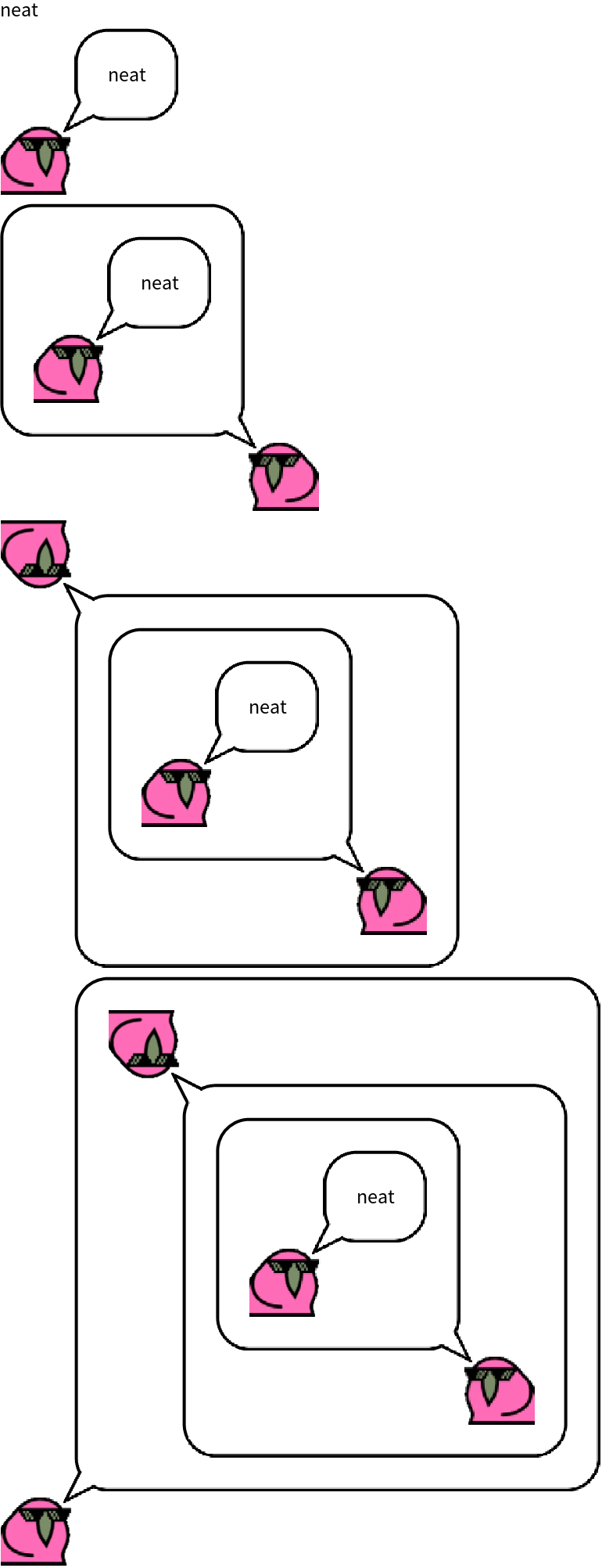Function Repository Resource:

FoldRightList

Compute a right-associated fold returning the list of intermediate results

Contributed by: Richard Hennigan (Wolfram Research)
 ResourceFunction["FoldRightList"][f,x,{e1,…,en-1,en}] gives {x,f[en,x],f[en-1,f[en,x]],…}. ResourceFunction["FoldRightList"][f,{e1,…,en-2,en-1,en}] gives {en,f[en-1,en],f[en-2,f[en-1,en]],…}. ResourceFunction["FoldRightList"][f] represents an operator form of ResourceFunction["FoldRightList"] that can be applied to expressions.

Details and Options

A right-associated fold is often called foldr.
With a length n list, ResourceFunction["FoldRightList"] generates a list of length n+1.
ResourceFunction["FoldRightList"][f,x,list] is effectively equivalent to FoldList[f[#2,#1]&,x,Reverse[list]].
ResourceFunction["FoldRightList"][f][list] is equivalent to ResourceFunction["FoldRightList"][f,list].
ResourceFunction["FoldRightList"][f][x,list] is equivalent to ResourceFunction["FoldRightList"][f,x,list].

Examples

Basic Examples (6)

Perform a right fold on a list:

 In:=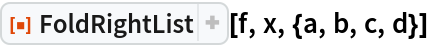Out=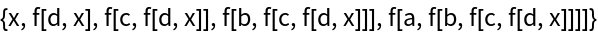Get cumulative sums of the elements of a list beginning from the end:

 In:=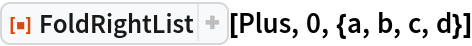Out=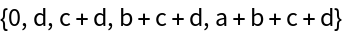Cumulative powers:

 In:=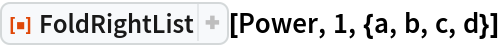Out=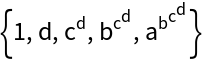Use the last element of the list as the starting value:

 In:=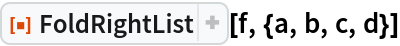Out=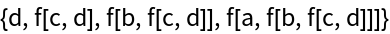Use the operator form of FoldRightList on one argument:

 In:=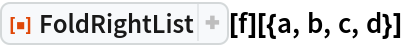Out=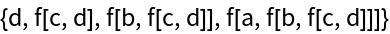Use the operator form of FoldRightList on two arguments:

 In:=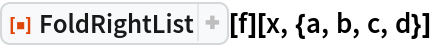Out=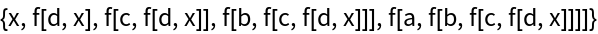Scope (1)

Perform a chain of cross products:

 In:=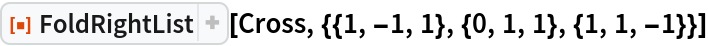Out=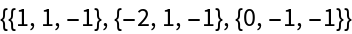Generalizations and Extensions (4)

The head need not be List:

 In:=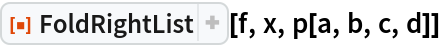Out=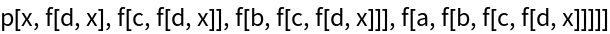Use Unevaluated to fold prior to evaluation:

 In:=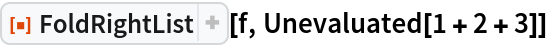Out=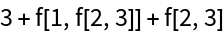Hold and fold with Hold:

 In:=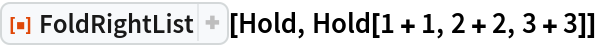Out=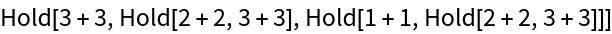Fold to the left:

 In:=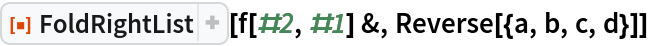Out=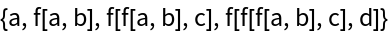Or equivalently:

 In:=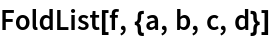Out=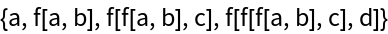Applications (6)

Form alternating sums:

 In:=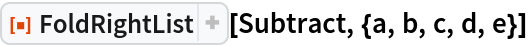Out=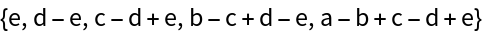Form continued fractions:

 In:=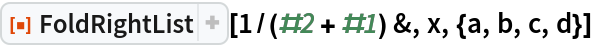Out=Build up a nested polynomial (Horner form):

 In:=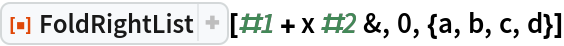Out=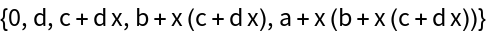Build up a binary tree:

 In:=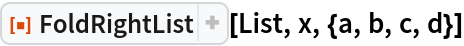Out=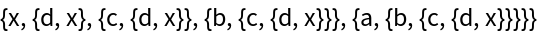Form a sequence of function compositions:

 In:=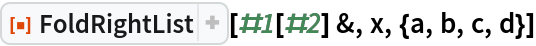Out=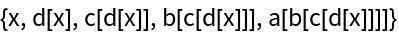Compare to using FoldList to form right compositions:

 In:=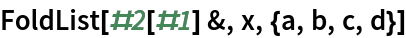Out=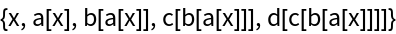Form compositions of subvalues:

 In:=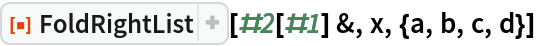Out=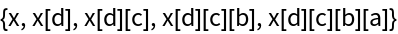Properties and Relations (7)

FoldRightList makes a list of length n+1:

 In:=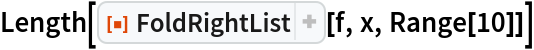Out=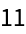Folding with an empty list does not apply the function at all:

 In:=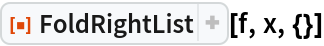Out=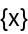The resource function FoldRight gives the last element of FoldRightList:

 In:=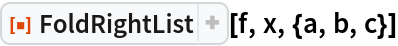Out=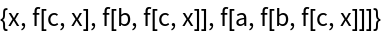In:=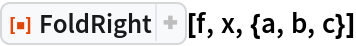Out=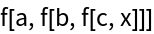Functions that ignore their first argument give the same result as NestList:

 In:=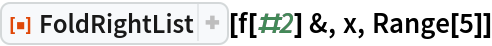Out=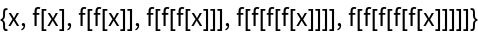In:=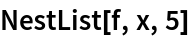Out=Compare FoldRightList to FoldList structurally:

 In:=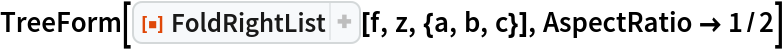Out=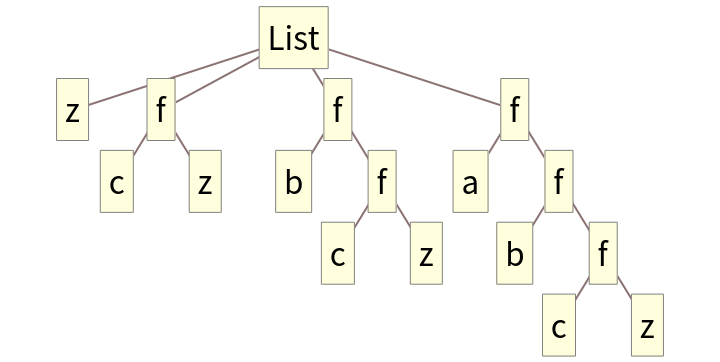In both cases, each item in the list is a subtree of the next:

 In:=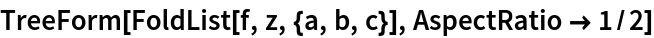Out=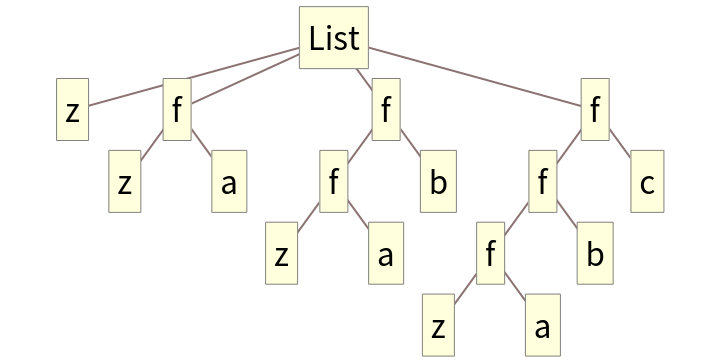The order of arguments passed to f are reversed compared to FoldList:

 In:=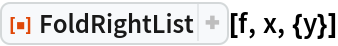Out=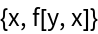In:=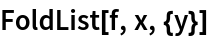Out=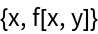FoldRightList takes elements starting on the right of the given list:

 In:=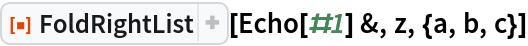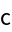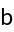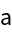Out=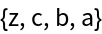Compare to FoldList:

 In:=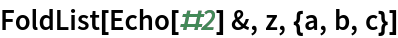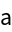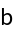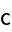Out=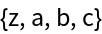Possible Issues (2)

FoldRightList[f,{}] evaluates to an empty list, without a last element:

 In:=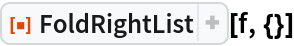Out=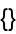Therefore, FoldRight[f,{}] is undefined:

 In:=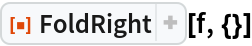Out=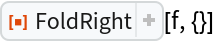Elements are taken in reverse order:

 In:=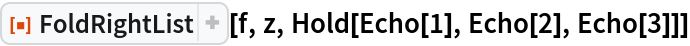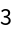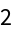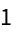Out=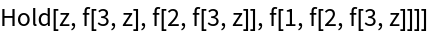Compare to a typical recursive definition of foldr:

 In:=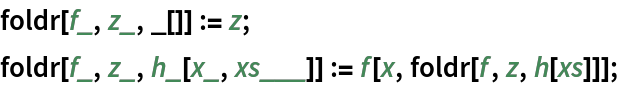In:=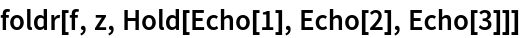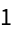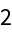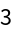Out=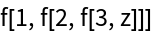This implementation allows FoldRightList to be tail recursive, which is far more efficient with respect to stack size:

 In:=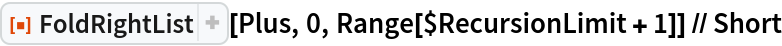Out=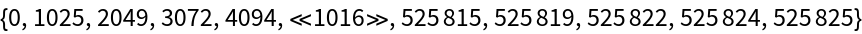Without tail recursion, the stack size would grow with respect to the size of the list:

 In:=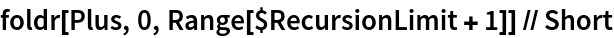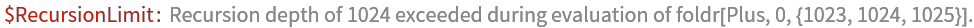Out=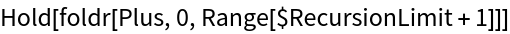Neat Examples (1)

Compute a four-fold bird fold for fun:

 In:=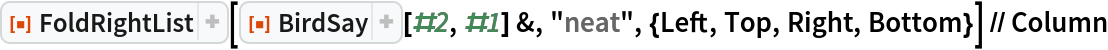Out=